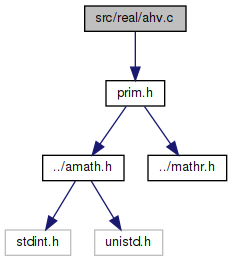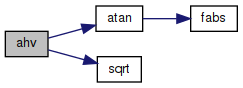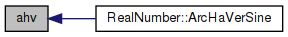amath  1.8.5 Simple command line calculator
ahv.c File Reference

Inverse haversed sine. More...

`#include "prim.h"`
Include dependency graph for ahv.c:Go to the source code of this file.

## Functions

double ahv (double x)
Inverse haversed sine. More...

## Detailed Description

Inverse haversed sine.

Definition in file ahv.c.

## ◆ ahv()

 double ahv ( double x )

Inverse haversed sine.

Definition at line 40 of file ahv.c.

References atan(), and sqrt().

Referenced by RealNumber::ArcHaVerSine().

41 {
42  double a = x - x * x;
43  double b = 2.0 * sqrt(a);
44  double c = 1.0 - 2.0 * x;
45  double d = atan(b / c);
46  return d;
47 }
double atan(double x)
Inverse tangent function.
Definition: atan.c:103
double sqrt(double x)
Square root function.
Definition: sqrt.c:119
Here is the call graph for this function:Here is the caller graph for this function: